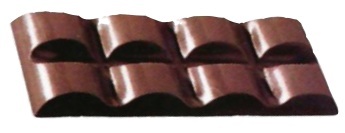Home | | Maths 5th Std | Compare fractions

# Compare fractions

Finds a number corresponding to a part of collection in the form of fractions. To identify the terms like numerator and denominator.

UNIT−6

FRACTIONSCompare fractions

Finds a number corresponding to a part of collection in the form of fractions

Situation

Radhika bought a chocolate bar, which is divided into 8 pieces. She wants to share it with his brother Tharun. She gave him 2 parts out of 8 parts. But he denied to havethat. He needed more. Then she gave him 3 out of 8 parts, which was also refused by him. So she finally decided to give him 4 out of 8 parts. Again, he was not willing to take. He wants more. But she explained him that she had given him equal share only.Consider the above situation. How will you express 2 out of 8 parts mathematically?

2/8 Isn’t it?

How will you express 3 out of 8 parts?

3/8 Isn’t it?

Here, 2/8 and 3/8 are fractions.

Fraction is a part of a whole or collection.

Example 6.1

Given below is a collection of 7 stars,How will you represent the shaded and unshaded stars given above as fractions?

Solution

Total stars = 7

Fraction of the shaded stars = 5/7

Fraction of the unshaded stars = 2/7

Try these

Try these

i. Represent the crossed out part as a fraction.ii. Represent the figures that are outside the circle as a fraction.To identify the terms like numerator and denominator.

Consider the same situation. In 2/8, the number above the line represents the numerator that is ‘2’ and the number below the line represents the denominator, that is ‘8’. The small line that is inbetween the top number and bottom number is the division bar.

Try these

i. In 3/7, 3 is the rumerator and 7 is the denominator.

ii. In 6/10, 6 is the rumerator and 10 is the denominator.

To compare different simple fractions

Consider the same situation

In the first sharing

Radhika’s share = 6 / 8

Tharun’s Share = 2 / 8.

Who gets more pieces of chocolate?

Radhika will get more pieces of chocolate.

How do you know that?

By comparing the numerator. Denominator is equal here in both the fractions.

Check the Numerator: 2 < 6 (or) 6 > 2.

Therefore,

2/8 < 6/8 (or) 6/8> 2/8

Example 6.2

Represent the following figures in fraction, compare them and say which is greater ?

Solution

Fraction represented in fig (i) is 3/5 and the fraction represented in fig (ii) is 2/5.As the denominators in both the fractions are equal, checking the numerator, we get, 3 > 2.

Therefore, 3/5 > 2/5

Example 6.3

Write the following figures in fractions and say which is smaller?Solution

Fraction represented in fig(i) is 3/4 .

Fraction represented in fig(ii) is 1/4 .

As the denominators in both the fractions are equal, checking the numerator, we get 1 < 3.

Therefore,

1/4 < 3/4

Tags : Fractions | Term 3 Chapter 6 | 5th Maths , 5th Maths : Term 3 Unit 6 : Fractions
Study Material, Lecturing Notes, Assignment, Reference, Wiki description explanation, brief detail
5th Maths : Term 3 Unit 6 : Fractions : Compare fractions | Fractions | Term 3 Chapter 6 | 5th Maths